Question

The function C(x)=−20x+1681 represents the cost to produce x items. What is the least number of items that can be produced so that the average cost is no more than $21? in progress 0 2 months 2021-08-04T19:02:37+00:00 1 Answers 1 views 0 ## Answers ( ) 1. Answer: The least number of items to produce is 41 Step-by-step explanation: Average Cost Given C(x) as the cost function to produce x items. The average cost is: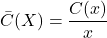The cost function is: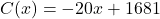And the average cost function is: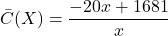We are required to find the least number of items that can be produced so the average cost is less or equal to$21.

We set the inequality: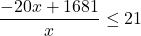Multiplying by x: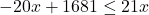Note we multiplied by x and did not flip the inequality sign because its value cannot be negative.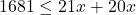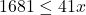Swapping sides and changing the sign: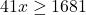Dividing by 41: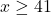The least number of items to produce is 41